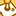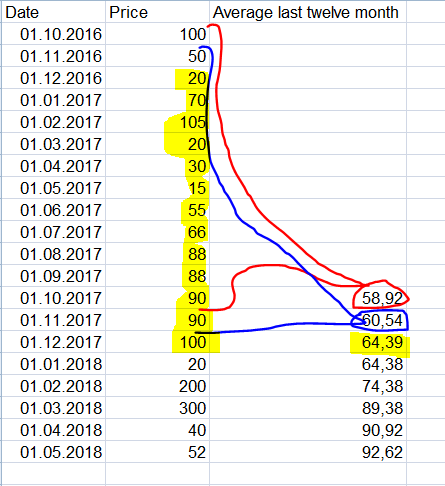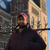# New to Qlik Sense

If you’re new to Qlik Sense, start with this Discussion Board and get up-to-speed quickly.

Announcements
cancel
Showing results for
Did you mean:Contributor III

## Average calculation

Hi friends,

I would like to calculate the average of the last 12 month (starting with 01.10.2017) and show them in a bar chart.

Can anyone help me with a formula to make it work like in my screenshot.

So the average from 01.10.2016 - 01.10.2017 would be 58,92 (red)

the average from 01.11.2016 - 01.11.2017 would be 60,54 (blue)

the average from 01.12.2016 - 01.12.2017 would be 54,39 (yellow)

and so on...Thank you for any help!

markus

1 Solution

Accepted SolutionsChampion

Hi,

try this:

=RangeAvg(Above(sum(Price),0,12))

if it gives you correct values, you can set an if condition to start the calculation from 01/10/2017

4 RepliesEmployee

Hi Markus,

Accumulative Sums

FYI, You are actually marking 13 months in Your illustration so You are actually doing calculation of 13 month rollling averages with those formulas which will throw You off if You compare to Sense formulas calculating 12 month rolling averages  - like Rangeavg(Above(sum(Price),0,12)).

Best Regards

LuckeChampion

Hi,

try this:

=RangeAvg(Above(sum(Price),0,12))

if it gives you correct values, you can set an if condition to start the calculation from 01/10/2017Contributor III
Author

Thank you Youssef!

It works exactly as I wanted to!

Best

MarkusChampion

you're welcome, don't forget to close the thread